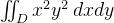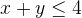# Calculating a double integral

#### Petrus

##### Well-known member
Calculate the double integral,
where D is the set of all points in the first quadrant which satisfies the inequality.
I am confused how to calculate the a,b $$\displaystyle \int_a^b$$ (I dont know what you call that in english)
Shall I do like this
$$\displaystyle y=4-x$$ and put it in the function so we get $$\displaystyle x^2(4-x)^2$$ and then?

#### tkhunny

##### Well-known member
MHB Math Helper
Re: multiple integrate

You're defining limits, not making a substitution.

$$\int\limits_{0}^{4}\int\limits_{0}^{4-y}x^{2}y^{2}dxdy$$

$$\int\limits_{0}^{4}y^{2}\left(\int\limits_{0}^{4-y}x^{2}dx\right) dy$$

#### MarkFL

Staff member
...
I am confused how to calculate the a,b $$\displaystyle \int_a^b$$ (I dont know what you call that in english)...
Hello Petrus,

Those values, $a$ and $b$, are referred to as the limits of integration. $a$ is the lower limit of integration, and $b$ is the upper limit of integration.

#### Petrus

##### Well-known member
I am having problem integrate $$\displaystyle \frac{1}{3}\int_0^4(4-y)^2y^2 dy$$
and I did not understand how we got this limits of integration.

#### MarkFL

Staff member
I am having problem integrate $$\displaystyle \frac{1}{3}\int_0^4(4-y)^2y^2 dy$$
and I did not understand how we got this limits of integration.
You have integrated the inner integral incorrectly. What is:

$$\displaystyle \int x^2\,dx$$ ?

As far as the limits go, can you describe the region over which you are integrating?

#### Petrus

##### Well-known member
You have integrated the inner integral incorrectly. What is:

$$\displaystyle \int x^2\,dx$$ ?

As far as the limits go, can you describe the region over which you are integrating?
$$\displaystyle \frac{x^3}{3}$$

- - - Updated - - -

ops I did not notice $$\displaystyle \frac{1}{3}\int_0^4(4-y)^3y^2 dy$$

#### MarkFL

Staff member
Yes, less the constant of integration. Do you see that you need $$\displaystyle (4-y)^3$$ in your remaining integrand?

edit: Yes, you caught the minor error!Now, do you know how to proceed?

#### Petrus

##### Well-known member
Yes, less the constant of integration. Do you see that you need $$\displaystyle (4-y)^3$$ in your remaining integrand?

edit: Yes, you caught the minor error!Now, do you know how to proceed?
Actually not. If I am honest I got so much problem with integration, I was trying integration by part but did not work well

#### MarkFL

Staff member
I think the most straightforward method here would be to expand the cubed binomial, then distribute the factor $y^2$. What is your integrand now?

#### Ackbach

##### Indicium Physicus
Staff member
I think the most straightforward method here would be to expand the cubed binomial, then distribute the factor $y^2$. What is your integrand now?
Or $u=4-y$ would make the algebra a little easier, as you'd only have to expand a quadratic instead of a cubic.

#### Petrus

##### Well-known member
I think the most straightforward method here would be to expand the cubed binomial, then distribute the factor $y^2$. What is your integrand now?
Do you mean like this?
$$\displaystyle \frac{1}{3}\int_0^4y^2(-y^3+12y^2-48y+64)$$

#### MarkFL

Staff member
Or $u=4-y$ would make the algebra a little easier, as you'd only have to expand a quadratic instead of a cubic.
Yes, great suggestion!

If you use this substitution Petrus, be mindful to rewrite the limits and differential in terms of the new variable, and use the rule:

$$\displaystyle \int_a^b f(x)\,dx=-\int_b^a f(x)\,dx$$

#### MarkFL

Staff member
Do you mean like this?
$$\displaystyle \frac{1}{3}\int_0^4y^2(-y^3+12y^2-48y+64)\,dy$$
Yes...I have added the missing differential.Now distribute the $y^2$, and integrate term by term to get the anti-derivative for use in the FTOC.

#### Petrus

##### Well-known member
Yes...I have added the missing differential.Now distribute the $y^2$, and integrate term by term to get the anti-derivative for use in the FTOC.
Is this what you mean $$\displaystyle \frac{1}{3}\int_0^4y^2 dy+\frac{1}{3}\int_0^4-y^3+12y^2-48y+64 dy$$

(Sorry for forgeting dy, this is something I always forget cause I think in my brain that I derivate dy

Edit: after I posted I start to notice that Is wrong.. you mean $$\displaystyle \frac{1}{3}\int_0^4y^2(-y^3)+\frac{1}{3}\int_0^4y^212y^2+\frac{1}{3}\int_0^4y^2(-48y)+\frac{1}{3}\int_0^4y^2*64$$

Last edited:

#### MarkFL

Staff member
No, that is an illegal move. You want to distribute the $y^2$ factor:

$$\displaystyle \frac{1}{3}\int_0^4(-y^5+12y^4-48y^3+64y^2)\,dy$$

#### Petrus

##### Well-known member
No, that is an illegal move. You want to distribute the $y^2$ factor:

$$\displaystyle \frac{1}{3}\int_0^4(-y^5+12y^4-48y^3+64y^2)\,dy$$
Yeah I notice when I submited, I just felt stupid that I posted it after I did submit because I obvious did not think correct.

#### MarkFL

Staff member
Yeah I notice when I submited, I just felt stupid that I posted it after I did submit because I obvious did not think correct.
Hey, we all make mistakes...some in haste. I've made my share for sure!Now, what is the anti-derivative you want to use in your application of the FTOC?

#### Petrus

##### Well-known member
Hey, we all make mistakes...some in haste. I've made my share for sure!Now, what is the anti-derivative you want to use in your application of the FTOC?
$$\displaystyle \frac{1}{3}\int_0^4(-y^5+12y^4-48y^3+64y^2)\,dy$$
I think you call it 'Second part' the one $$\displaystyle \int_a^bf(x)=F(b)-F(a)$$
our anti derivate is $$\displaystyle \frac{-y^6}{6}+\frac{12y^5}{5}-\frac{48y^4}{4}+\frac{64y^3}{3}$$
So now we got $$\displaystyle \frac{1}{3}[\frac{-y^6}{6}+\frac{12y^5}{5}-\frac{48y^4}{4}+\frac{64y^3}{3}]_0^4 =\frac{1}{3}(\frac{1024}{15}-0)=\frac{1024}{45}$$

#### MarkFL

Staff member
Yes, good work!I was taught to call it the anti-derivative form of the fundamental theorem of calculus. I notice many now call it the second theorem. Whatever you call it though, it gives us a powerful method for evaluating definite integrals.#### Petrus

##### Well-known member
Yes, great suggestion!

If you use this substitution Petrus, be mindful to rewrite the limits and differential in terms of the new variable, and use the rule:

$$\displaystyle \int_a^b f(x)\,dx=-\int_b^a f(x)\,dx$$
if $$\displaystyle u=4-y$$ then is $$\displaystyle y=4-u$$
so we got $$\displaystyle \frac{1}{3}\int_4^0u^3(4-u)^2 du$$ and with that rule we got $$\displaystyle -\frac{1}{3}\int_0^4u^3(4-u)^2 du$$ Is this correct?

#### MarkFL

Staff member
if $$\displaystyle u=4-y$$ then is $$\displaystyle y=4-u$$
so we got $$\displaystyle \frac{1}{3}\int_4^0u^3(4-u)^2$$ and with that rule we got $$\displaystyle -\frac{1}{3}\int_0^4u^3(4-u)^2$$ Is this correct?
Not quite...with:

$$\displaystyle u=4-y\,\therefore\,du=-dy$$

Notice this negative sign on the differential gets brought out front (this is one reason it is important to always include the differential in your integrations), and so rewriting everything in terms of the new variable $u$, we get:

$$\displaystyle -\frac{1}{3}\int_4^0u^3(4-u)^2\,du=\frac{1}{3}\int_0^4u^3(4-u)^2\,du$$

#### Petrus

##### Well-known member
Not quite...with:

$$\displaystyle u=4-y\,\therefore\,du=-dy$$

Notice this negative sign on the differential gets brought out front (this is one reason it is important to always include the differential in your integrations), and so rewriting everything in terms of the new variable $u$, we get:

$$\displaystyle -\frac{1}{3}\int_4^0u^3(4-u)^2\,du=\frac{1}{3}\int_0^4u^3(4-u)^2\,du$$
I still dont understand why 1/3 got negative.

#### MarkFL

Staff member
I still dont understand why 1/3 got negative.
Because $dy=-du$ and the negative sign represents the constant $-1$ and can be brought out in front of the integral as a factor.

#### Petrus

##### Well-known member
Because $dy=-du$ and the negative sign represents the constant $-1$ and can be brought out in front of the integral as a factor.
Thanks Mark and Ackback!I now got same answer answer and integrade in two wayThe only thing I did not understand is how we got this limits of integration. for x we got from 0 to 4-y and for y we got from 0 to 4 I did not understand how you get that.

#### Ackbach

##### Indicium Physicus
Staff member
Thanks Mark and Ackback!I now got same answer answer and integrade in two wayThe only thing I did not understand is how we got this limits of integration. for x we got from 0 to 4-y and for y we got from 0 to 4 I did not understand how you get that.
Because the original limits are $x$-values. When you do a $u$-substitution, you can either write the limits as $u$-values (by substituting into the $u=4-x$, in your case), or you can write the new limits as $u(0)$ to $u(4)$. Either way, the rule is as follows for $u$-substitutions:

1. For definite integrals, you must translate the integrand, the differential, and the limits. You can perform the entire integral in the $u$-domain, if you like.
2. For indefinite integrals, you must translate the integrand and the differential into the $u$-domain. However, once you have an antiderivative computed, you must go back to $x$'s, since that is what you were given.